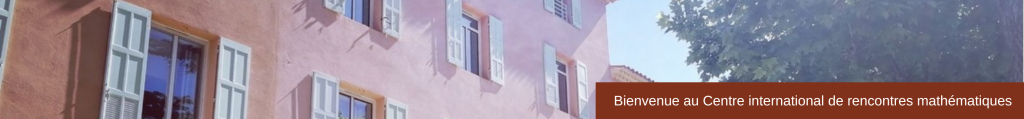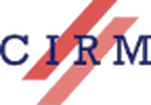RESEARCH IN PAIRS

Models of Group Schemes of Diagonalizable Group Schemes
January 9 – 13, 2017

 Let S = Spec(R), where R is a discrete valuation ring with residue field of positive. We would like to classify finite and flat group schemes over S of order a power of p which have diagonalizable generic fiber. Moreover we want see if one can extend Kummer theory to this kind of group schemes. This work could be useful to study the specialization, from zero to positive characteristic, of torsors under commutative groups. And it can be also some applications in the context of essential dimension, an invariant which gives the number of parameters to define a generic torsor. Participants Matthieu Romagny (Université de Rennes 1)Dajano Tossici (Université de Bordeaux) Sponsor